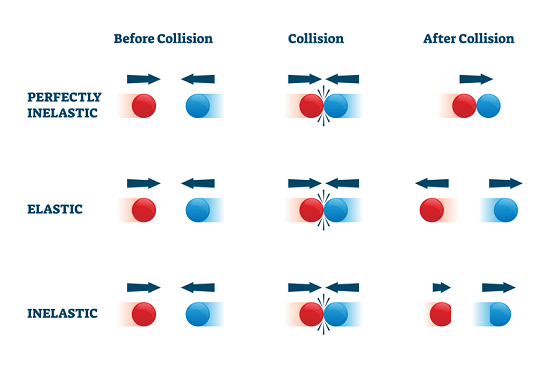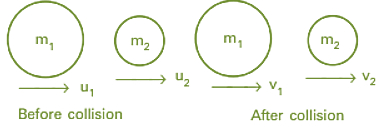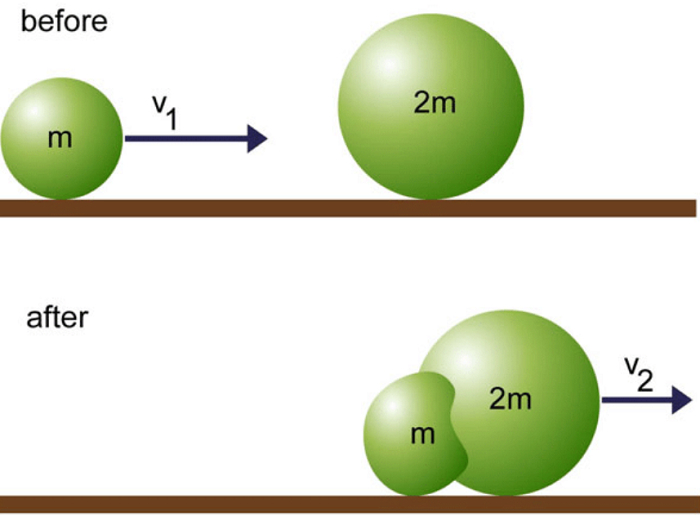# Elastic and Inelastic Collisions In One and Two Dimensions

## Introduction

In our daily life, we experience many kinds of collisions. For example, in cricket, a ball collides with the bat. The direction of the ball and bat is towards each other before the collision and after the collision the energy, speed, and direction of the ball change. In a collision, the interaction between the particles is for a short interval of time but the applied force is relatively very strong on each other. Collisions may be elastic or inelastic.

## What is Elastic Collision?

During the elastic collision, the kinetic energy or linear momentum of the bodies which collide is constant or in simple words conserved which means there is exactly no loss of kinetic energy. The collisions of the particles are elastic.## What is an Inelastic Collision?

During the inelastic collision, the kinetic energy of the system, there is a loss of energy which means energy is not conserved and the particles stick with each other and then move. Momentum remains conserved. Initial Kinetic energy is always greater than final kinetic energy.

## Types of Collision

Mainly there are two types of collision: One is elastic collision and the other is Inelastic collision. In the first case, the kinetic energy is constant whereas in the second case the kinetic energy is not conserved.

## Law of Conservation of Linear Momentum

As we know, momentum is measured to be the multiplication of mass and the velocity of an object. Now from the law of conservation of linear momentum, if one object collides with another body, the total momentum of an object held before a collision is the same or equal to the total momentum of an object held after a collision.

Let, velocities initially before the collision be $\mathrm{u_1}$ and $\mathrm{u_2}$. and velocities final after the collision is $\mathrm{v_{1}}$ and $\mathrm{v_{2}}$. Mass is constant, so the mass remains constant after the collision. A force has an equal and opposite reaction.

$$\mathrm{F_1=-F_2}$$

$$\mathrm{m_1 a_1=-m_2 a_2}$$

$$\mathrm{m_1\frac{(v_{1}-u_{1})}{t}=-m_2\frac{(v_{2}-u_{2})}{t}}$$

Time t has been canceled

$$\mathrm{m_1 v_{1}-m_1 u_1=-m_2 v_{2}+m_2 u_2}$$

$$\mathrm{m_1 v_{1}-m_1 u_1=m_2 u_{2}-m_2 v_2}$$

$$\mathrm{m_1 v_{1}+m_2 v_2=m_2 u_{2}+m_1 u_1}$$

Or $$\mathrm{m_1 u_{1}+m_2 u_2=m_1 v_{1}+m_2 v_2}$$

Hence, the total momentum held before the collision is equal to or the same as the total momentum held after the collision.

## Elastic Collision in One DimensionFig:1 Elastic collision in one dimension

Suppose two bodies or objects having mass $\mathrm{m_1}$ and $\mathrm{m_2}$ move with straight or uniform line initially of velocities $\mathrm{u_{1}}$ and $\mathrm{u_{2}}$ as ($\mathrm{u_{1}}$ is greater than $\mathrm{u_{2}}$) and after the collision of bodies their velocities become $\mathrm{v_1}$ and $\mathrm{v_2}$ as ($\mathrm{v_2}$ is greater than $\mathrm{v_1}$)

According to the law of conservation of momentum,

$$\mathrm{m_1 u_{1}+m_2 u_2=m_1 v_{1}+m_2 v_2}$$

$$\mathrm{m_1 u_{1}-m_1 v_1=m_2 v_{2}-m_2 u_2}$$

$$\mathrm{m_1(u_{1}-v_1)=m_2 (v_2-u_2) \:\:\: ….(1)}$$

By the law of conservation of energy, KE is conserved

$$\mathrm{\frac{1}{2}m_1u_1^{2}+\frac{1}{2}m_1u_2^{2}=\frac{1}{2}m_2v_1^{2}+\frac{1}{2}m_2v_2^{2}}$$

$$\mathrm{\frac{1}{2}m_1u_1^{2}-\frac{1}{2}m_1v_1^{2}=\frac{1}{2}m_2v_2^{2}-\frac{1}{2}m_2u_2^{2}}$$

$$\mathrm{\frac{1}{2}m_1(u_1^{2}-v_1^{2})=\frac{1}{2}m_2(v_2^{2}-u_2^{2})}$$

$$\mathrm{m_1(u_1^{2}-v_1^{2})=m_2(v_2^{2}-u_2^{2})\:\:\:\:….(2)}$$

Dividing eq (2) by (1)

$$\mathrm{\frac{m_1(u_1^{2}-v_1^{2})}{m_1(u_1-v_1)}=\frac{m_2(v_2^{2}-u_2^{2})}{m_2(v_2-u_2)}}$$

$$\mathrm{\frac{(u_1 +v_1 )((u_(1 )-v_1)}{(u_(1 )-v_1)}=\frac{(v_2 -u_2 )(v_2+u_2)}{(v_2-u_2)}}$$

(As the same values canceled out)

$$\mathrm{(u_1+v_1)=(v_2+u_2)}$$

$$\mathrm{(u_1 -u_2)=(v_2-v_1)}$$

Therefore relative velocity before the collision $\mathrm{(u_1-u_2)}$ is equal to relative collision after collision $\mathrm{(v_2-v_1)}$.

## Elastic Collision in Two Dimension

From the conservation of energy,

Total Kinetic Energy(KE) held before the collision is always the same or equal to the total Kinetic Energy(KE) held after the collision.

$$\mathrm{\frac{1}{2}m_1u_1^{2}+\frac{1}{2}m_1u_2^{2}=\frac{1}{2}m_2v_1^{2}+\frac{1}{2}m_2v_2^{2}}$$

(1/2 will be canceled out, so we have)

$$\mathrm{m_1 u_1^{2}+m_1 u_2^{2}=m_2 v_1^{2}+m_2 v_2^{2}}$$

As linear momentum is constant,

Total linear momentum held before the collision is the same as total linear momentum held after the collision. For horizontal components

$$\mathrm{m_1 u_{1}+m_2 u_2=m_1 v_{1}\:cos \theta +m_2 v_2\:cos \theta}$$

For vertical components

$$\mathrm{0=m_1 v_{1} sin \theta +m_2 v_2 sin \theta}$$

The four unknown quantities, which are $\mathrm{m_1,m_2,v_1,u_2,\theta}$, by measuring one of the four unknown quantities the value of the other three unknown quantities can be solved.

## Inelastic Collision in One Dimension

In an inelastic collision, bodies stick to each other and move in the same direction as speed. Momentum is conserved but the kinetic energy may vary and change to different types of energy. Due to bodies stick each other, the conservation of momentum is,

$$\mathrm{m_1 u_{1}+m_2 u_2=(m_1+m_2)v}$$

Here the final velocity becomes v and we find the value of v as:

$$\mathrm{v=\frac{m_1 u_{1}+m_2 u_2}{(m_1+m_2)}}$$

Also, the kinetic energy lost is given by,

$$\mathrm{KE=\frac{1}{2}m_1u_{2}-\frac{1}{2}(m_1+m_2)v_2}$$

## Inelastic Collision in Two Dimension

As we know total linear momentum of the system of any particles will remain constant which means total initial momentum is equal to total final momentum.

As we have,

$$\mathrm{m_1 u_{1}+m_2 u_2=m_1 v_{1}\:cos \theta +m_2 v_2\:cos\theta}$$

$$\mathrm{0=m_1 v_{1} sin \theta-m_2 v_2 sin\theta}$$### Inelastic collision in two dimension

As the bodies are stuck to each other,

$$\mathrm{m_1 u_{1}cos \theta +m_2 u_2=(m_1+m_2)v\:cos \theta}$$

Therefore the momentum on the left-hand side is equal to the linear momentum right side which is the final momentum. So this is the equation for perfectly inelastic collision. After the collision velocities become the same. so final velocity is denoted as v.

### Examples of one and two dimension collision

Some examples related to the collision are:

• When you hit the basketball on the ground, it bounces back to your side. In this case, the kinetic energy remains constant, which means this example is of elastic collision.

• The collision between the small particles and the atoms is also an example of elastic collision.

• A boy playing football on the ground is an example of an inelastic collision.

• A muddy ball thrown to the wall sticks to it, this is for inelastic collision.

• If two cars hit each other is an example of an inelastic collision.

• If a ball of clay is dropped at some height it sticks also due to inelastic collision.

## Conclusion

This tutorial on collision can be seen as an application of the conservation of linear momentum. Various technologies in physics have used these concepts to understand more about collision

## FAQs

Q1. A bus hitting a tree is an example of which type of collision?

Ans. In this example, the kinetic energy(KE) is not constant, so it is an Inelastic collision.

Q2. From inelastic collision, is Kinetic energy constant?

Ans. No, kinetic energy(KE) can never be conserved or constant in an inelastic collision.

Q3. Is momentum constant in the case of an inelastic collision?

Ans. Yes, momentum is constant in an inelastic collision.

Q4. Define conservation of momentum.

Ans. From the law of conservation of momentum, the sum of the momentum of collision held before is the same as that of the total momentum of collision held after.

Q5. Some bodies stick with other bodies, which type of collision is this?

Ans. Inelastic collision